# All Classes and Courses

## Find the resources you need to learn about computational fields and further your understanding of Wolfram technologies.

Click any button to browse all catalog resources in a particular area or use the filters to refine your search. The catalog includes free, self-paced, interactive courses, standalone video lessons, video courses and scheduled instructor-led courses as well as archived and special events. Learn about the different course types.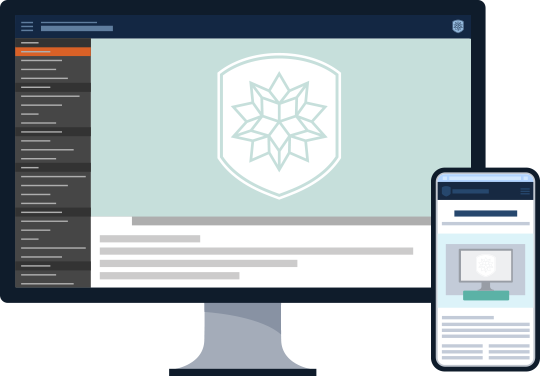Refine & Sort Showing filtered results
• Course Type
• Difficulty
• Certification
• Time to Complete
• Clear options
• Sort by
• Clear options

42 results

Hybrid Computing Quick Start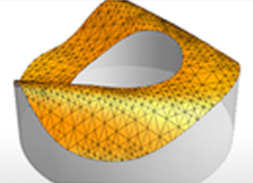##### Hybrid Computing Quick Start
###### Video Lesson | FREE

One of the unique strengths of the Wolfram Language is its ability to take advantage of symbolic computation and exact numbers when solving numerical problems. This ability allows users to find the right answer in many cases where purely approximate methods would fail and opens up a world of powerful hybrid symbolic-numeric methods. In this class you'll get a look under the hood at some of the ways the numerics of the Wolfram Language benefit from its symbolics and how method selection is automatically inferred. The class is for those who have a basic familiarity with numeric and symbolic computation in the Wolfram Language.

Hypothesis Testing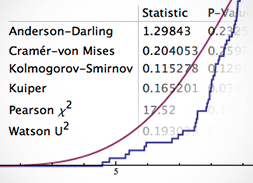##### Hypothesis Testing
###### Video Lesson | FREE

The Wolfram Language includes a framework for hypothesis testing. This class explores parametric and nonparametric tests of location, variance or scale, as well as distributional goodness of fit. This class assumes basic familiarity with the Wolfram Language and statistics concepts.

Introduction to Discrete Mathematics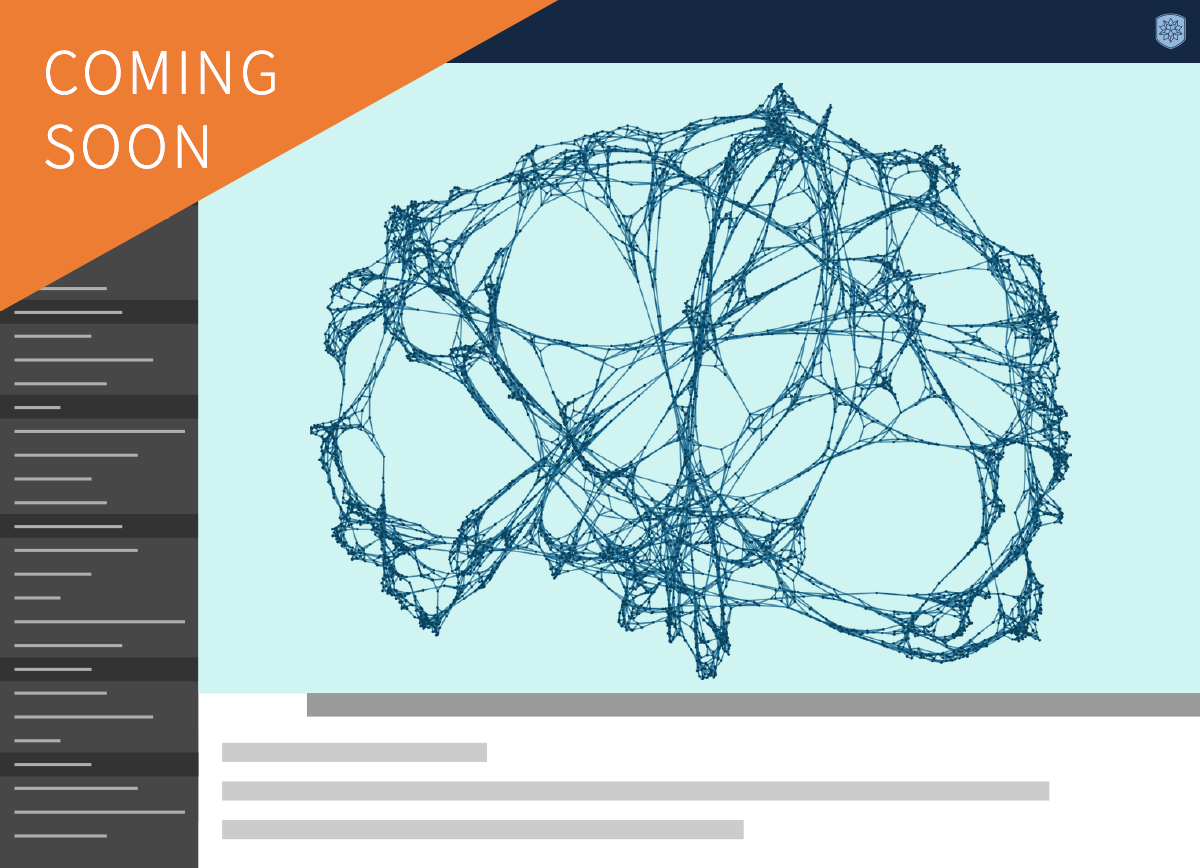##### Introduction to Discrete Mathematics
###### Interactive Course | FREE

Requirements: This course requires no prior knowledge of Mathematica or Wolfram Language. Prerequisites for discrete mathematics include algebra, geometry and precalculus.

Certification Levels: CompletionLevel 1

Get an introduction to notions of logic, discrete structures, counting, algorithms, proofs, recurrences and graphs.

Introduction to Multivariable Calculus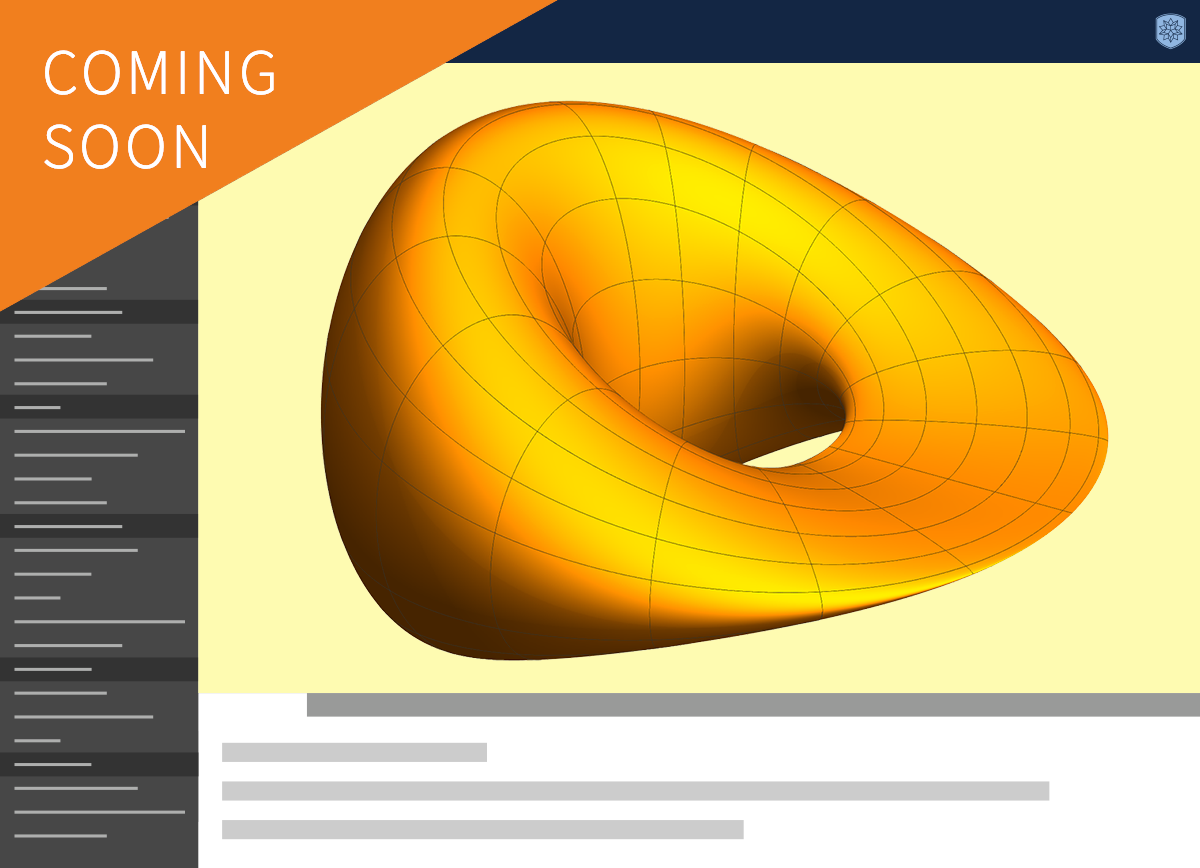##### Introduction to Multivariable Calculus
###### Interactive Course | FREE

Requirements: This course requires basic working knowledge of Mathematica or Wolfram Language. The prerequisite for multivariable calculus is single-variable calculus.

Certification Levels: CompletionLevel 1

This comprehensive multivariable calculus course extends the notions of limits, derivatives and integrals to higher dimensions. It also considers constrained and unconstrained optimization problems.

Mathematica and Wolfram Language for
Mathematics Research and Study (Study Group Sessions)##### Mathematica and Wolfram Language for Mathematics Research and Study (Study Group Sessions)
###### Archived Event | FREE

This Daily Study Group is designed especially for mathematics graduate students and research professionals but covers topics of interest to an even wider audience. This Study Group will help you make the most of the built-in functionality available in Wolfram Language for advanced studies and research in mathematics—from special functions and visualization to solving differential equations, as well as number theory, combinatorics, differential geometry and more. Participants are encouraged to prepare for this Study Group by attending a Hands-on Start to Mathematica training tutorial.

Mathematica for the Calculus Classroom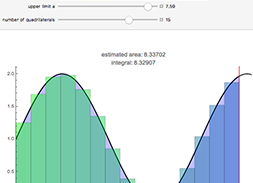##### Mathematica for the Calculus Classroom
###### Video Lesson | FREE

Mathematica is the educational software standard for the first-year calculus classroom, allowing students to visualize concepts and develop skills essential for other STEM classes. This video demonstrates easy ways to integrate interactive activities, visualizations and other teaching tools into first-year calculus lessons with the use of Wolfram technologies. Learn how natural language input, a predictive interface and point-and-click palettes allow students to begin using Mathematica and the Wolfram Language without learning syntax.

Model Fitting and Analysis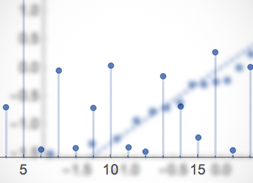##### Model Fitting and Analysis
###### Video Lesson | FREE

This class explores the built-in Wolfram Language framework for linear and nonlinear regression, logit, probit and generalized linear models, as well as optimization functions, linear algebra functions and a package for analysis of variance (ANOVA). The class assumes basic familiarity with the Wolfram Language and statistics concepts.

Modeling with Statistical Distributions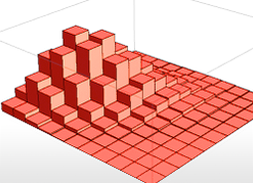##### Modeling with Statistical Distributions
###### Video Lesson | FREE

The Wolfram Language has the largest collection of parametric and nonparametric distributions, supporting high-level functions for probability and statistics. This class demonstrates application of these functions for modeling and analysis in statistics. Presentation topics also include automated probability and statistics superfunctions, streamlined processes for parameter estimation and goodness-of-fit testing, working with built-in distributions or creating your own from data or other distributions, and statistical visualization functionality.

New in Wolfram Language 13.1 Webinar Series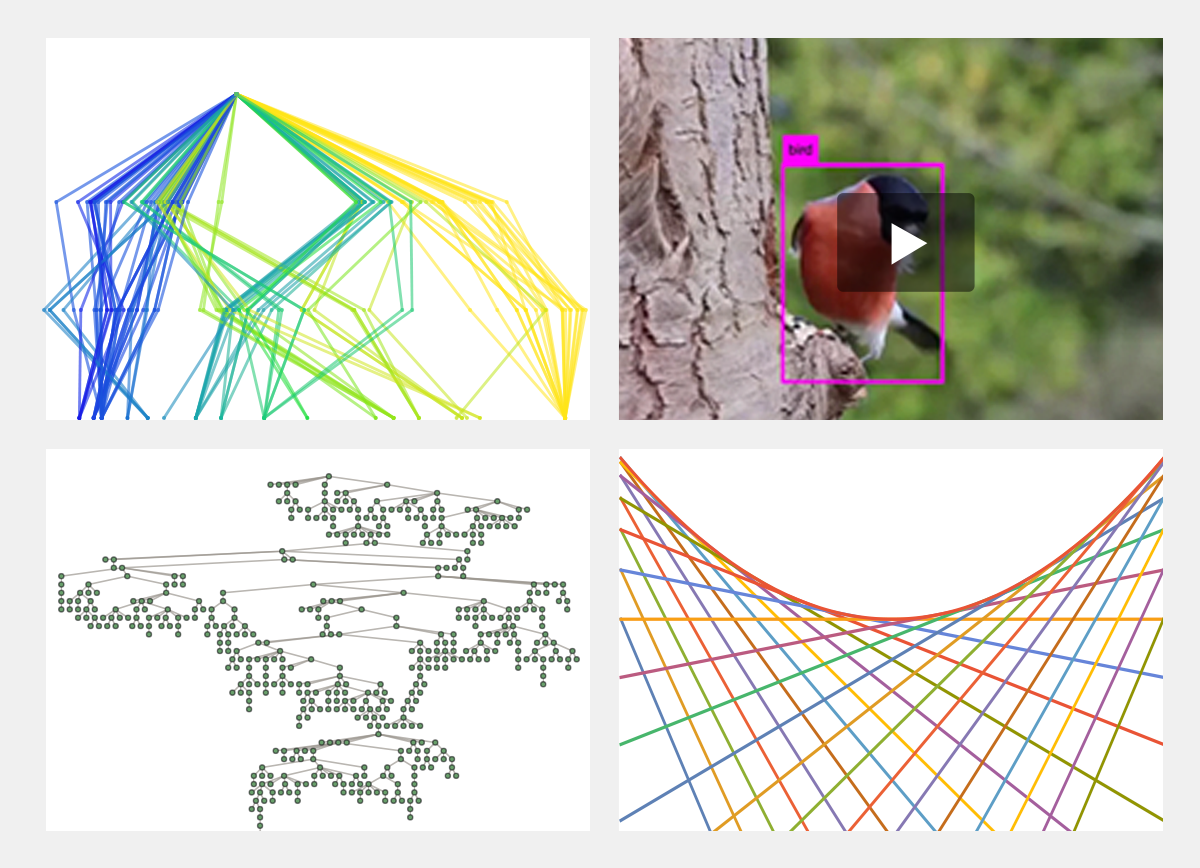##### New in Wolfram Language 13.1 Webinar Series
###### Archived Event | FREE

Learn about what's new in the latest release of Wolfram Language in this three-part webinar series. The first session includes an overview of the latest functionality in Version 13.1 as well as a presentation on the new notebook toolbar and other front end improvements. The second webinar covers what's new in calculus and algebra, with a focus on fractional calculus. The third webinar in the series shares updates in machine learning, new video functions and the tree construct.

###### Course Overview
• Video 181 minutes
• Video 275 minutes
• Video 388 minutes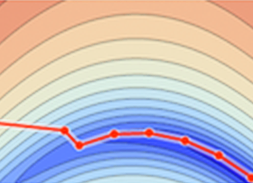###### Video Lesson | FREE

Learn best practices for using advanced numeric functionality in the Wolfram Language. Topics include techniques for combining numeric functions, advanced numeric features and understanding precision and accuracy. This class is for those who have experience with Wolfram Language numeric functions and are ready for advanced control and customization features.

Solving ODEs and PDEs with Wolfram Language (Study Group Sessions)##### Solving ODEs and PDEs with Wolfram Language (Study Group Sessions)
###### Archived Event | FREE

This Daily Study Group covers techniques for solving ordinary differential equations (ODEs) and partial differential equations (PDEs) using Wolfram Language. It introduces the built-in function DSolve for finding symbolic solutions to differential equations and the built-in function NDSolve, a general numerical differential equation solver. Topics covered include the numerical method of lines, the finite element method (FEM), the use and construction of meshes, boundary value problems and eigenvalue problems.

Solving PDEs with Symbolics and Numerics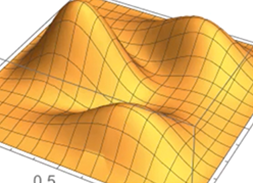##### Solving PDEs with Symbolics and Numerics
###### Video Course | FREE

This video course provides a thorough introduction to solving partial differential equations (PDEs) in the Wolfram Language both symbolically and numerically. You'll learn how to solve boundary value problems for classical PDEs and obtain solutions for the Schrödinger and other modern PDEs using the Wolfram Language function DSolve and its numerical counterpart NDSolve. You'll also discover how to solve PDEs over regions, find eigenvalues and eigenfunctions over regions with DEigensystem and NDEigensystem and use the latest Wolfram Language functionality to create better PDE models and gain a deeper understanding of your physics and engineering designs.

###### Course Overview
• Video 126 minutes
• Video 225 minutes

Statistical Analysis with Wolfram Language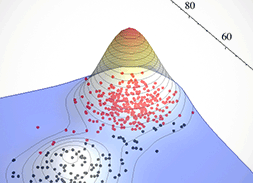##### Statistical Analysis with Wolfram Language
###### Video Course | FREE

Analyze your data using a framework of model fitting and statistical analysis built into the Wolfram Language. Whether it is basic descriptive and exploratory statistics or advanced modeling with statistical distributions, you can follow this video course to gain an understanding of the statistical functionality available in the Wolfram Language. Topics covered include descriptive measures, transformations, basic clustering, statistical distributions, parameter estimation and hypothesis testing. Advanced topics on using optimization functions, linear algebra functions, analysis of variance (ANOVA) and generalized logit and probit linear models will also be explored.

###### Course Overview
• Video 111 minutes
• Video 224 minutes
• Video 314 minutes
• Video 417 minutes
• Video 534 minutes

Statistical Distributions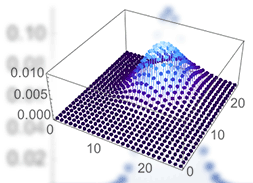##### Statistical Distributions
###### Video Lesson | FREE

The Wolfram Language includes extensive coverage for statistical distributions. This class explores the properties of derived distributions, probability distributions, mixture distributions and more. Other topics include random number generation and parameter estimation. This class assumes basic familiarity with the Wolfram Language and statistics concepts.

Symbolic and Numeric Computation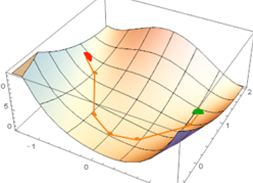##### Symbolic and Numeric Computation
###### Video Course | FREE

This video course illuminates the powerful combination of symbolic and numeric computing available in the Wolfram Language. See how the numerical functions and methods in the Wolfram Language benefit from symbolic computation with exact numbers, where purely approximate methods may fail. Tips and techniques for using multiple numerical functions together, along with advanced numeric features, are included.

###### Course Overview
• Video 130 minutes
• Video 233 minutes

Wavelet Analysis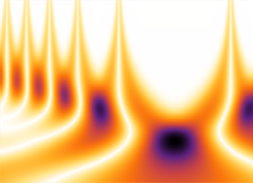##### Wavelet Analysis
###### Video Course | FREE

Understand your data better with wavelet analysis. These videos offer an in-depth introduction to wavelets, starting from basic concepts and continuing into advanced methods and uses. You'll learn the essentials of wavelet decomposition of signals—including different types of wavelet transforms, common algorithms, visualizations and interpretation of results—with a focus on general applications in signal processing and time series analysis. Examples are presented from financial analysis, edge detection, denoising of images, data compression and more.

###### Course Overview
• Video 149 minutes
• Video 252 minutes

Wavelet Applications##### Wavelet Applications
###### Video Lesson | FREE

This video class presents examples from a variety of applications, including financial time series, edge detection and denoising of images, thresholding, image and data compression and image fusion. Familiarity with Fourier transforms and data smoothing methods is recommended for this class.

Wavelet Concepts##### Wavelet Concepts
###### Video Lesson | FREE

Wavelets decompose a signal into approximations and details at different scales, making them useful for applications such as data compression, detecting features and removing noise from signals. This class explains some of the theory behind continuous, discrete and stationary wavelet transforms and demonstrates how the Wolfram Language and its built-in functions can be used to construct, compute, visualize and analyze wavelet transforms and related functions. Familiarity with Fourier transforms and data smoothing methods is recommended for this class.

## Course Type

• Interactive Courses
• Video Lessons
• Video Courses
• Instructor-led Courses
• Archived and Special Events

### Interactive Courses

Also known as MOOCs (massive open online courses), these courses are hosted on the Wolfram Cloud and allow you to interactively explore concepts using Wolfram Language functionality.

• Self-paced with progress tracking

• Include video lessons, exercises and problems, quizzes, exams and a scratch notebook

• Sharable completion certificates available for all courses

• Wolfram Level 1 proficiency certifications available for select courses

### Video Lessons

Short recorded lessons that provide limited instruction on a computational topic or for using Wolfram tech.

• Quick-start videos

• Lessons from content experts

• A wide variety of beginner-level lessons

• Free to watch

### Video Courses

Video series that build on preceding lessons to provide comprehensive instruction.

• Each video course features a playlist of sequential lessons

• Recorded by Wolfram certified instructors

• Comprehensive coverage of a particular topic

• Free to watch

### Instructor-led Courses

Scheduled as online and in-person classes, these courses provide comprehensive instruction guided by a live instructor.

• Registration required to reserve your seat

• Taught by Wolfram certified instructors

• Opportunity to pose live questions to experts in the room

• Course completion certificates available

### Archived and Special Events

Presentations by Wolfram developers, content experts and instructors.

• Webinars on special topics and new release functionality

• Livecoding sessions

• Wolfram Daily Study Groups

• Free to watch